# HC Verma solutions for Class 11, Class 12 Concepts of Physics Vol. 1 chapter 5 - Newton's Laws of Motion [Latest edition]

#### Chapters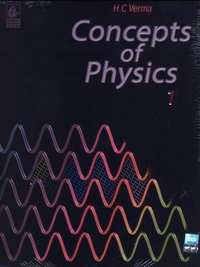## Chapter 5: Newton's Laws of Motion

short answers [Pages 76 - 77]

### HC Verma solutions for Class 11, Class 12 Concepts of Physics Vol. 1 Chapter 5 Newton's Laws of Motion short answers [Pages 76 - 77]

short answers | Q 1 | Page 76

The apparent weight of an object increases in an elevator while accelerating upward. A person sells peanuts using a beam balance in an elevator. Will he gain more if the elevator is accelerating up?

short answers | Q 2 | Page 76

A boy puts a heavy box of mass M on his head and jumps from the top of a multi-storied building to the ground. What is the force exerted by the box on the boy's head during his free fall? Does the force greatly increase during the period he balances himself after striking the ground?

short answers | Q 3 | Page 76

A person drops a coin. Describe the path of the coin as seen by the person if he is in (a) a car moving at constant velocity and (b) in a free falling elevator.

short answers | Q 4 | Page 76

Is it possible for a particle to describe a curved path if no force acts on it? Does your answer depend on the frame of reference chosen to view the particle?

short answers | Q 5 | Page 76

You are travelling in a car. The driver suddenly applies the brakes and you are pushed forward. Why does this happen?

short answers | Q 6 | Page 76

It is sometimes heard that the inertial frame of reference is only an ideal concept and no such inertial frame actually exists. Comment.

short answers | Q 7 | Page 76

An object is placed far away from all the objects that can exert force on it. A frame of reference is constructed by taking the origin and axes fixed in this object. Will the frame be necessarily inertial?

short answers | Q 8 | Page 76

The figure shows a light spring balance connected to two blocks of mass 20 kg each. The graduations in the balance measure the tension in the spring. (a) What is the reading of the balance? (b) Will the reading change if the balance is heavy, say 2.0 kg? (c) What will happen if the spring is light but the blocks have unequal masses?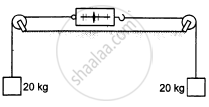short answers | Q 9 | Page 77

The acceleration of a particle is zero, as measured from an inertial frame of reference. Can we conclude that no force acts on the particle?

short answers | Q 10 | Page 77

Suppose you are running fast in a field and suddenly find a snake in front of you. You stop quickly. Which force is responsible for your deceleration?

short answers | Q 11 | Page 77

If you jump barefoot on a hard surface, your legs are injured. But they are not injured if you jump on a soft surface like sand or pillow. Why?

short answers | Q 12 | Page 77

According to Newton's third law, each team pulls the opposite team with equal force in a tug of war. Then, why does one team win and the other lose?

short answers | Q 13 | Page 77

A spy jumps from an airplane with his parachute. The spy accelerates downward for some time when the parachute opens. The acceleration is suddenly checked and the spy slowly falls to the ground. Explain the action of the parachute in checking the acceleration.

short answers | Q 14 | Page 77

Consider a book lying on a table. The weight of the book and the normal force by the table in the book are equal in magnitude and opposite in direction. Is this an example of Newton's third law?

short answers | Q 15 | Page 77

Two blocks of unequal masses are tied by a spring. The blocks are pulled stretching the spring slightly and the system is released on a frictionless horizontal platform. Are the forces due to the spring on the two blocks equal and opposite? If yes, is it an example of Newton's third law?

short answers | Q 16 | Page 77

When a train starts, the head of a standing passenger seems to be pushed backward. Analyse the situation from the ground frame. Does it really go backward? Coming back to the train frame, how do you explain the backward movement of the head on the basis of Newton's laws?

short answers | Q 17 | Page 77

A plumb bob is hung from the ceiling of a train compartment. If the train moves with an acceleration 'a' along a straight horizontal track , the string supporting the bob makes an angle tan−1 (a/g) with the normal to the ceiling. Suppose the train moves on an inclined straight track with uniform velocity. If the angle of incline is tan−1 (a/g), the string again makes the same angle with the normal to the ceiling. Can a person sitting inside the compartment tell by looking at the plumb line whether the train is accelerating on a horizontal straight track or moving on an incline? If yes, how? If not, then suggest a method to do so.

MCQ [Pages 77 - 78]

### HC Verma solutions for Class 11, Class 12 Concepts of Physics Vol. 1 Chapter 5 Newton's Laws of Motion MCQ [Pages 77 - 78]

MCQ | Q 1 | Page 77

A body of weight w1 is suspended from the ceiling of a room by a chain of weight w2. The ceiling pulls the chain by a force.

•  w1

• w2

• w1 + w2

• $\frac{w_1 + w_2}{2}$

MCQ | Q 2 | Page 77

When a horse pulls a cart, the force that helps the horse to move forward is the force exerted by

• the cart on the horse

• the ground on the horse

• the ground on the cart

•  the horse on the ground

MCQ | Q 3 | Page 77

A car accelerates on a horizontal road due to the force exerted by.

• the engine of the car

• the driver of the car

• the earth

MCQ | Q 4 | Page 77

A block of mass 10 kg is suspended from two light spring balances, as shown in the following figure.• Both the scales will read 10 kg.

• Both the scales will read 5 kg.

• The upper scale will read 10 kg and the lower zero.

• The readings may be anything but their sums will be 10 kg.

MCQ | Q 5 | Page 77

A block of mass m is placed on a smooth inclined plane of inclination θ with the horizontal. The force exerted by the plane on the block has a magnitude.

•  mg

• mg/cosθ

• mg cosθ

•  mg tanθ

MCQ | Q 6 | Page 77

A block of mass m is placed on a smooth wedge of inclination θ. The whole system is accelerated horizontally so that the block does not slip on the wedge. The force exerted by the wedge on the block has a magnitude.

• mg

• mg/cosθ

• mg cosθ

• mg tanθ

MCQ | Q 7 | Page 77

Neglect the effect of rotation of the earth. Suppose the earth suddenly stops attracting objects placed near its surface. A person standing on the surface of the earth will.

• fly up

• slip along the surface

• fly along a tangent to the earth's surface

• remain standing

MCQ | Q 8 | Page 77

Three rigid rods are joined to form an equilateral triangle ABC of side 1 m. Three particles carrying charges 20 μC each are attached to the vertices of the triangle. The whole system is at rest in an inertial frame. The magnitude of the resultant force on the charged particle at A is.

• zero

• 3.6 N

• 3.6sqrt3 N

• 7.2 N

MCQ | Q 9 | Page 78

A force F1 acts on a particle accelerating it from rest to a velocity v. Force F1 is then replaced by F2 which decelerates the particle to rest.

• F1 must be equal to F2.

• F1 may be equal to F2.

• F1 must be unequal to F2

MCQ | Q 10 | Page 78

Two objects A and B are thrown upward simultaneously with the same speed. The mass of A is greater than that of B. Suppose the air exerts a constant and equal force of resistance on the two bodies.

• The two bodies will reach the same height.

• A will go higher than B.

• B will go higher than A.

•  Any of the above three may happen depending on the speed with which the objects are thrown.

MCQ | Q 11 | Page 78

A smooth wedge A is fitted in a chamber hanging from a fixed ceiling near the earth's surface. A block B placed at the top of the wedge takes time T to slide down the length of the wedge. If the block is placed at the top of the wedge and the cable supporting the chamber is broken at the same instant, the block will.

•  take a time longer than T to slide down the wedge

• take a time shorter than T to slide down the wedge

• remain at the top of the wedge

• jump off the wedge

MCQ | Q 12 | Page 78

In an imaginary atmosphere, the air exerts a small force F on any particle in the direction of the particle's motion. A particle of mass m projected upward takes time t1 in reaching the maximum height and t2 in the return journey to the original point. Then.

• t1 < t2

•  t1 > t2

• t1 = t2

• the relation between t1 and t2 depends on the mass of the particle

MCQ | Q 13 | Page 78

A person standing on the floor of an elevator drops a coin. The coin reaches the floor of the elevator in time t1 if the elevator is stationary and in time t2 if it is moving uniformly. Then

• t1 = t2

• t1 < t2

•  t1 > t2

• t1 < t2 or t1 > t2 depending on whether the lift is going up or down.

MCQ | Q 14 | Page 78

A free 238U nucleus kept in a train emits an alpha particle. When the train is stationary, a nucleus decays and a passenger measures that the separation between the alpha particle and the recoiling nucleus becomes x at time t after the decay. If the decay takes place while the train is moving at a uniform velocity v, the distance between the alpha particle and the recoiling nucleus at a time t after the decay, as measured by the passenger, is

• x + v t

• x − v t

•  x

• depends on the direction of the train

MCQ [Pages 78 - 79]

### HC Verma solutions for Class 11, Class 12 Concepts of Physics Vol. 1 Chapter 5 Newton's Laws of Motion MCQ [Pages 78 - 79]

MCQ | Q 1 | Page 78

A reference frame attached to the earth
(a) is an inertial frame by definition
(b) cannot be an inertial frame because the earth is revolving around the sun
(c) is an inertial frame because Newton's laws are applicable in this frame
(d) cannot be an inertial frame because the earth is rotating about its axis.

MCQ | Q 2 | Page 78

A particle stays at rest as seen in a frame. We can conclude that
(a) the frame is inertial
(b) resultant force on the particle is zero
(c) the frame may be inertial but the resultant force on the particle is zero
(d) the frame may be non-inertial but there is a non-zero resultant force

MCQ | Q 3 | Page 78

A particle is found to be at rest when seen from a frame S1 and moving with constant velocity when seen from another frame S2. Mark out the possible options.
(a) Both the frames are inertial.
(b) Both the frames are non-inertial.
(c) S1 is inertial and S2 is non-inertial.
(d) S1 is non-inertial and S2 is inertial

MCQ | Q 4 | Page 78

The figure shows the displacement of a particle going along the X-axis as a function of time. The force acting on the particle is zero in the region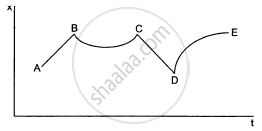(a) AB
(b) BC
(c) CD
(d) DE

MCQ | Q 5 | Page 78

The figure shows a heavy block kept on a frictionless surface and being pulled by two ropes of equal mass m. At t = 0, the force on the left rope is withdrawn but the force on the right end continues to act. Let F1 and F2 be the magnitudes of the forces exerted by right rope and the left rope on the block, respectively.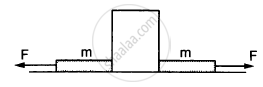• F1 = F2 = F for t < 0

• F1 = F2 = F + mg for t < 0

• F1 = F, F2 = F for t > 0

• F1 < F, F2 = F for t > 0

MCQ | Q 6 | Page 79

The force exerted by the floor of an elevator on the foot of a person is more than the weight of the person if the elevator is
(a) going up and slowing down
(b) going up and speeding up
(c) going down and slowing down
(d) going down and speeding up

MCQ | Q 7 | Page 79

If the tension in the cable supporting an elevator is equal to the weight of the elevator, the elevator may be
(a) going up with increasing speed
(b) going down with increasing speed
(c) going up with uniform speed
(d) going down with uniform speed

MCQ | Q 8 | Page 79

A particle is observed from two frames S1 and S2. Frame S2 moves with respect to S1with an acceleration a. Let F1 and F2 be the pseudo forces on the particle when seen from S1 and S2, respectively. Which of the following is not possible?

• F1 = 0, F2 ≠ 0

• F1 ≠ 0, F2 = 0

• F1 ≠ 0, F2 ≠ 0

• F1 = 0, F2 = 0

MCQ | Q 9 | Page 79

A person says that he measured the acceleration of a particle to be non-zero even though no force was acting on the particle.

• He is a liar.

• His clock might have run slow.

• His metre scale might have been longer than the standard.

• He might have used a non-inertial frame.

Exercise [Pages 79 - 83]

### HC Verma solutions for Class 11, Class 12 Concepts of Physics Vol. 1 Chapter 5 Newton's Laws of Motion Exercise [Pages 79 - 83]

Exercise | Q 1 | Page 79

A block of mass 2 kg placed on a long frictionless horizontal table is pulled horizontally by a constant force F. It is found to move 10 m in the first seconds. Find the magnitude of F.

Exercise | Q 2 | Page 79

car moving at 40 km/hr is to be stopped by applying brakes in the next 4 m. If the car weighs 2000 kg, what average force must be applied to stop it?

Exercise | Q 3 | Page 79

In a TV picture tube, electrons are ejected from the cathode with negligible speed and they attain a velocity of 5 × 106 m/s in travelling one centimetre. Assuming straight-line motion, find the constant force exerted on the electrons. The mass of an electron is 9.1 × 10−31 kg.

Exercise | Q 4 | Page 79

A block of mass 0.2 kg is suspended from the ceiling by a light string. A second block of mass 0.3 kg is suspended from the first block by another string. Find the tensions in the two strings. Take g = 10 m/s2.

Exercise | Q 5 | Page 79

Two blocks of equal mass m are tied to each other through a light string. One of the blocks is pulled along the line joining them with a constant force F. Find the tension in the string joining the blocks.

Exercise | Q 6 | Page 79

A particle of mass 50 g moves in a straight line. The variation of speed with time is shown in the following figure. Find the force acting on the particle at t = 2, 4 and 6 seconds.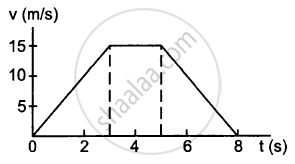Exercise | Q 7 | Page 79

Two blocks A and B of mass mA and mB , respectively, are kept in contact on a frictionless table. The experimenter pushes block A from behind, so that the blocks accelerate. If block A exerts force F on block B, what is the force exerted by the experimenter on block A?

Exercise | Q 8 | Page 79

Raindrops of radius 1 mm and mass 4 mg are falling with a speed of 30 m/s on the head of a bald person. The drops splash on the head and come to rest. Assuming equivalently that the drops cover a distance equal to their radii on the head, estimate the force exerted by each drop on the head.

Exercise | Q 9 | Page 79

A particle of mass 0.3 kg is subjected to a force F = −kx with k = 15 N/m. What will be its initial acceleration if it is released from a point x = 20 cm?

Exercise | Q 10 | Page 79

Both the springs shown in the following figure are unstretched. If the block is displaced by a distance x and released, what will be the initial acceleration?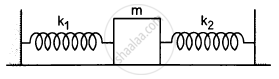Exercise | Q 11 | Page 79

A small block B is placed on another block A of mass 5 kg and length 20 cm. Initially, the block B is near the right end of block A (In the following Figure). A constant horizontal force of 10 N is applied to the block A. All the surfaces are assumed frictionless. Find the time that elapses before block B separates from A.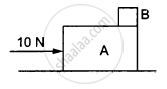Exercise | Q 12 | Page 79

A man has fallen into a ditch of width d and two of his friends are slowly pulling him out using a light rope and two fixed pulleys as shown in the following figure. Show that the force (assumed equal for both the friends) exerted by each friend on the road increases as the man moves up. Find the force when the man is at a depth h.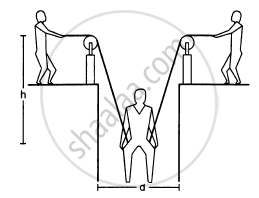Exercise | Q 13 | Page 80

The elevator shown in the following figure is descending with an acceleration of 2 m/s2. The mass of the block A is 0.5 kg. What force is exerted by the block A on the block B?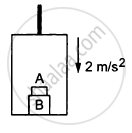Exercise | Q 14 | Page 80

A pendulum bob of mass 50 g is suspended from the ceiling of an elevator. Find the tension in the string if the elevator (a) goes up with acceleration 1.2 m/s2, (b) goes up with deceleration 1.2 m/s2, (c) goes up with uniform velocity, (d) goes down with acceleration 1.2 m/s2, (e) goes down with deceleration 1.2 m/s2 and (f) goes down with uniform velocity.

Exercise | Q 15 | Page 80

A person is standing on a weighing machine placed on the floor of an elevator. The elevator starts going up with some acceleration, moves with uniform velocity for a while and finally decelerates to stop. The maximum and the minimum weights recorded are 72 kg and 60 kg, respectively. Assuming that the magnitudes of acceleration and deceleration are the same, find (a) the true weight of the person and (b) the magnitude of the acceleration. Take g = 9.9 m/s2.

Exercise | Q 16 | Page 80

Find the reading of the spring balance shown in the following figure. The elevator is going up with an acceleration g/10, the pulley and the string are light and the pulley is smooth.Exercise | Q 17 | Page 80

A block of 2 kg is suspended from a ceiling by a massless spring of spring constant k = 100 N/m. What is the elongation of the spring? If another 1 kg is added to the block, what would be the further elongation?

Exercise | Q 18 | Page 80

Suppose the ceiling in the previous problem is that of an elevator which is going up with an acceleration of 2.0 m/s2. Find the elongation.

Exercise | Q 19 | Page 80

The force of buoyancy exerted by the atmosphere on a balloon is B in the upward direction and remains constant. The force of air resistance on the balloon acts opposite the direction of velocity and is proportional to it. The balloon carries a mass M and is found to fall to the earth's surface with a constant velocity v. How much mass should be removed from the balloon so that it may rise with a constant velocity v?

Exercise | Q 20 | Page 80

An empty plastic box of mass m is found to accelerate up at the rate of g/6 when placed deep inside water. How much sand should be put inside the box so that it may accelerate down at the rate of g/6?

Exercise | Q 21 | Page 80

A force $\vec{F} = \vec{v} \times \vec{A}$ is exerted on a particle in addition to the force of gravity, where $\vec{v}$ is the velocity of the particle and $\vec{A}$ is a constant vector in the horizontal direction. With what minimum speed, a particle of mass m be projected so that it continues to move without being defelected and with a constant velocity?

Exercise | Q 22 | Page 80

In a simple Atwood machine, two unequal masses m1 and m2 are connected by a string going over a clamped light smooth pulley. In a typical arrangement (In the following figure), m1 = 300 g and m2 = 600 g. The system is released from rest. (a) Find the distance travelled by the first block in the first two seconds; (b) find the tension in the string; (c) find the force exerted by the clamp on the pulley.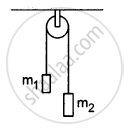Exercise | Q 23 | Page 80

Consider the Atwood machine of the previous problem. The larger mass is stopped for a moment, 2.0 s after the system is set into motion. Find the time that elapses before the string is tight again.

Exercise | Q 24 | Page 80

In the following figure shows a uniform rod of length 30 cm and mass 3.0 kg. The strings shown in the figure are pulled by constant forces of 20 N and 32 N. Find the force exerted by the 20 cm part of the rod on the 10 cm part. All the surfaces are smooth and the strings and the pulleys are light.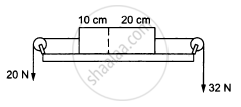Exercise | Q 25 | Page 81

Consider the situation shown in the following figure  All the surfaces are frictionless and the string and the pulley are light. Find the magnitude of acceleration of the two blocks.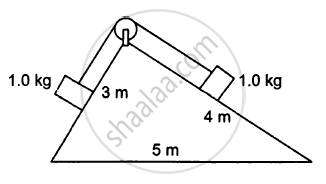Exercise | Q 26 | Page 81

A constant force F = m2g/2 is applied on the block of mass m1 as shown in the following figure. The string and the pulley are light and the surface of the table is smooth. Find the acceleration of m1.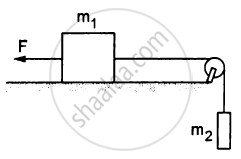Exercise | Q 27 | Page 81

In the following figure, m1 = 5 kg, m2 = 2 kg and F = 1 N. Find the acceleration of either block. Describe the motion of m1 if the string breaks but F continues to act.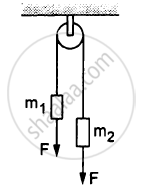Exercise | Q 28 | Page 81

Let m1 = 1 kg, m2 = 2 kg and m3 = 3 kg in the following figure. Find the accelerations of m1, m2 and m3. The string from the upper pulley to m1 is 20 cm when the system is released from rest. How long will it take before m1 strikes the pulley?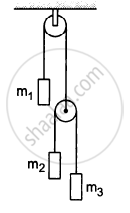Exercise | Q 29 | Page 81

In the previous problem, suppose m2 = 2.0 kg and m3 = 3.0 kg. What should be the mass m, so that it remains at rest?

Exercise | Q 30 | Page 81

Calculate the tension in the string shown in the following figure. The pulley and the string are light and all the surfaces are frictionless. Take g = 10 m/s2.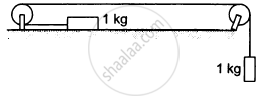Exercise | Q 31 | Page 81

Consider the situation shown in the following figure. Both the pulleys and the string are light and all the surfaces are frictionless. (a) Find the acceleration of the mass M; (b) find the tension in the string; (c) calculate the force exerted by the clamp on the pulley A in the figure.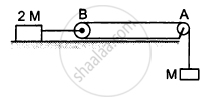Exercise | Q 32 | Page 81

Find the acceleration of the block of mass M in the situation shown in the following figure. All the surfaces are frictionless and the pulleys and the string are light.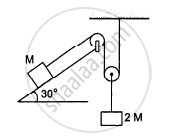Exercise | Q 33 | Page 82

Find the mass M of the hanging block in the following figure that will prevent the smaller block from slipping over the triangular block. All the surfaces are frictionless and the strings and the pulleys are light.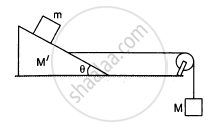Exercise | Q 34 | Page 82

Find the acceleration of the blocks A and B in the three situations shown in the following figure.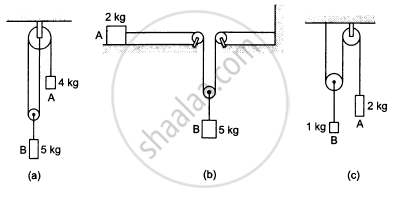Exercise | Q 35 | Page 82

Find the acceleration of the 500 g block in the following figure.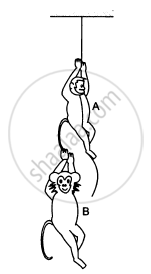Exercise | Q 36 | Page 82

A monkey of mass 15 kg is climbing a rope fixed to a ceiling. If it wishes to go up with an acceleration of 1 m/s2, how much force should it apply on the rope? If the rope is 5 m long and the monkey starts from rest, how much time will it take to reach the ceiling?

Exercise | Q 37 | Page 82

A monkey is climbing on a rope that goes over a smooth light pulley and supports a block of equal mass at the other end in the following figure. Show that whatever force the monkey exerts on the rope, the monkey and the block move in the same direction with equal acceleration. If initially both were at rest, their separation will not change as time passes.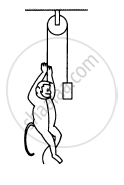Exercise | Q 38 | Page 82

The monkey B, shown in the following figure, is holding on to the tail of monkey A that is climbing up a rope. The masses of monkeys A and B are 5 kg and 2 kg, respectively. If A can tolerate a tension of 30 N in its tail, what force should it apply on the rope in order to carry monkey B with it? Take g = 10 m/s2.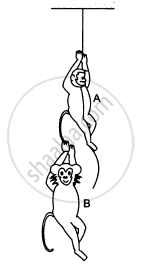Exercise | Q 39 | Page 82

In the following figure shows a man of mass 60 kg standing on a light weighing machine kept in a box of mass 30 kg. The box is hanging from a pulley fixed to the ceiling by a light rope, the other end of which is held by the man himself. If the man manages to keep the box at rest, what is the weight recorded on the machine? What force should he exert on the rope to record his correct weight on the machine?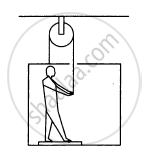Exercise | Q 40 | Page 83

A block A can slide on a frictionless incline of angle θ and length l, kept inside an elevator going up with uniform velocity v in the following figure. Find the time taken by the block to slide down the length of the incline if it is released from the top of the incline.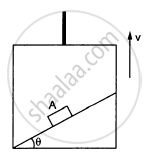Exercise | Q 41 | Page 83

A car is speeding up a horizontal road with acceleration a. Consider the following situations in the car: (i) A ball suspended from the ceiling by a string is maintaining a constant angle with the vertical. Find this angle. (ii) A block is kept on a smooth incline and does not slip on the incline. Find the angle of the incline with the horizontal.

Exercise | Q 42 | Page 83

A block is kept on the floor of an elevator at rest. The elevator starts descending with an acceleration of 12 m/s2. Find the displacement of the block during the first 0.2 s after the start. Take g = 10 m/s2.

## Chapter 5: Newton's Laws of Motion## HC Verma solutions for Class 11, Class 12 Concepts of Physics Vol. 1 chapter 5 - Newton's Laws of Motion

HC Verma solutions for Class 11, Class 12 Concepts of Physics Vol. 1 chapter 5 (Newton's Laws of Motion) include all questions with solution and detail explanation. This will clear students doubts about any question and improve application skills while preparing for board exams. The detailed, step-by-step solutions will help you understand the concepts better and clear your confusions, if any. Shaalaa.com has the CBSE Class 11, Class 12 Concepts of Physics Vol. 1 solutions in a manner that help students grasp basic concepts better and faster.

Further, we at Shaalaa.com provide such solutions so that students can prepare for written exams. HC Verma textbook solutions can be a core help for self-study and acts as a perfect self-help guidance for students.

Concepts covered in Class 11, Class 12 Concepts of Physics Vol. 1 chapter 5 Newton's Laws of Motion are Lubrication - (Laws of Motion), Law of Conservation of Linear Momentum and Its Applications, Aristotle’s Fallacy, The Law of Inertia, Newton’s Second Law of Motion, Newton's Third Law of Motion, Equilibrium of a Particle, Common Forces in Mechanics, Circular Motion and Its Characteristics, Solving Problems in Mechanics, Static and Kinetic Friction, Laws of Friction, Inertia, Intuitive Concept of Force, Dynamics of Uniform Circular Motion - Centripetal Force, Examples of Circular Motion (Vehicle on a Level Circular Road, Vehicle on a Banked Road), Newton's First Law of Motion, Rolling Friction, Introduction of Motion in One Dimension, Conservation of Momentum.

Using HC Verma Class 12 solutions Newton's Laws of Motion exercise by students are an easy way to prepare for the exams, as they involve solutions arranged chapter-wise also page wise. The questions involved in HC Verma Solutions are important questions that can be asked in the final exam. Maximum students of CBSE Class 12 prefer HC Verma Textbook Solutions to score more in exam.

Get the free view of chapter 5 Newton's Laws of Motion Class 12 extra questions for Class 11, Class 12 Concepts of Physics Vol. 1 and can use Shaalaa.com to keep it handy for your exam preparation